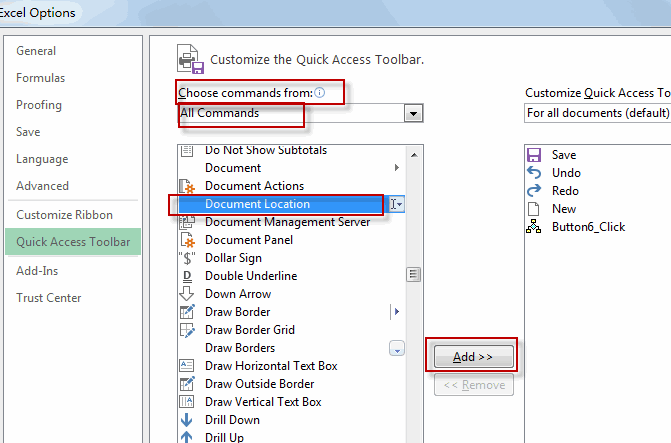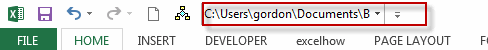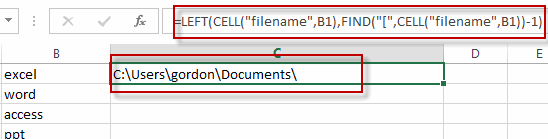# Get Workbook Path Only

This post will guide you how to get the current workbook path in Excel. How do I insert workbook path only into a cell with a formula in Excel.

## Get workbook path with Document Location

You can get the workbook path from the document location feature, and copy the location to a cell. Do the following steps:

#1 go to File tab, and click Options menu from the popup menu list. And the Excel Options dialog will open.

#2 click Quick Access Toolbar option, and select All commands from the drop down list of the Choose commands from. And then select Document Location value, click Add button to add it into Quick Access Toolbar.#3 click OK button. The workbook path is displayed in the Quick Access toolbar. Press Ctrl +C to copy it.## Get workbook path with Formula

You can also use a formula based on the LEFT function, the Cell function, and the FIND function to get workbook path only in Excel.  Just like this:

`=LEFT(CELL("filename",B1),FIND("[",CELL("filename",B1))-1)`

Type this formula into a cell and then press Enter key.Let’s see how this formula works:

The Cell function will be used to get the full name and path of the workbook file.

The Find function will return the location number of the first left square bracket.

The left function will extract the workbook path based on the number returned by the Find function.

### Related Functions

• Excel Find function
The Excel FIND function returns the position of the first text string (substring) from the first character of the second text string.The FIND function is a build-in function in Microsoft Excel and it is categorized as a Text Function.The syntax of the FIND function is as below:= FIND  (find_text, within_text,[start_num])…
• Excel LEFT function
The Excel LEFT function returns a substring (a specified number of the characters) from a text string, starting from the leftmost character.The LEFT function is a build-in function in Microsoft Excel and it is categorized as a Text Function.The syntax of the LEFT function is as below:= LEFT(text,[num_chars])…
• Excel CELL function
The Excel CELL function returns information about the formatting, location, size, or contents of a cell.The syntax of the CELL function is as below:= CELL (info_type,[reference])…